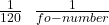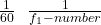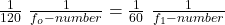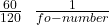## A properly exposed photograph is taken at f/16 and 1/120s. What lens opening is required if the shutter speed is 1/60s

Question

A properly exposed photograph is taken at f/16 and 1/120s. What lens opening is required if the shutter speed is 1/60s

in progress 0
5 months 2021-08-10T05:54:53+00:00 1 Answers 15 views 0

## Answers ( )

f / 8

Explanation:

The f-number is the ratio of the lens’s focal length to its diameter and is a measure of the intensity of the light reaching the CCD. The smaller the greater the intensity of the light

I = D / f

f-number = f / D

I = 1 / f-number

the energy deposited in the CCD is proportional to the intensity of the light and the exposure time (Δt)

E = I Δt

E = 1 /f-number   Δt

in the exercise they indicate that the system is well exposed (the image is sharp and clear) for fo-number = f / 16

Δt = exposure time = 1/120 s

E =indicate that the exposure time has been changed to Δt = 1/60 s, which should be the f₁-number

E =the energy deposited must be the same per location we can equal the expressionsf₁-number =f₁-number = ½  16

f₁-number  = 8

so the system should be set to f / 8